# 3D Programming In Turbo Pascal

## Introduction

In this tutorial I am going to explain to you as simply as possible how to program objects so that they seem to have a 3rd Dimension. I am not going to go into any kind of shading, filling, mapping etc. as this tutorial is for those who have never tried programming in 3D before. The more you know about Pascal the better the understanding of this tutorial, although I have tried to make this very easy to follow and understand by all. Why Pascal ? Well simply because Pascal is may main programming language, also Pascal is a good leader to the more popular languages of C and C++, where they are less structured and therefore harder to explain with. I will be using Pascal in all my examples, having a Pascal compiler (Turbo Pascal 7.0 for DOS is the best!) would be useful if you wish to learn from this tutorial.

The examples needed for this tutorial are below.

The examples themselves are only to illustrate the possibilities with the knowledge of how to write in 3D. This tutorial with give you the know how and therefore after going through this you should be able to do any type of 3D rotating and shading, with any objects you wish.

There are several ways in which programmers can make 3D objects, although the method that I am going to explain to you is not the absolute fastest (it is still very fast!) it is the most easiest to grasp and understand, and once you have got the idea, confidence and theory sorted on this method I am sure you will be able to work out other ways in which you can do the same task.

## The Idea Behind The Theory.

The actual theory does include mainly maths, don't get put off now !! I will explain exactly what each bit does, and why we use it, but for you to truly understand and remember what 3D programming is all about, it will take your commitment to practice and practice.

Now the real theory know how - programming 3 dimensional objects is based on the theories of circles. (drawing them! Now you are confused! Read on.....)

## How To Program In Three Dimensions

Firstly lets think about the drawing of a single pixel on a screen. The pixel has 2 co-ordinates, an X co-ordinate, and a Y co-ordinate.

Simply you would place the pixel N pixels along the horizontal line (X co-ordinate) and N pixels along the vertical line (Y co-ordinate), very simple. Put this information into a computer and the computer will display a pixel at those co-ordinates. BIG DEAL!!. But when you want something in 3D, that pixel or object of some kind, does not just have an X and Y co-ordinate, but also a Z co-ordinate, which represents the depth in which the pixel is (Not real !! but with the right know how you can make it seem to the computer user that the pixel has depth)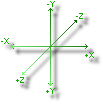Getting the minus and plus signs right is important (you don't want the object to go the wrong way round ! :-( )
So now we know that every pixel or point must have an X, Y and Z co-ordinate, this does not automatically make the object 3D, now we need the maths.

Before we start the maths we need to consider what we are going to do with the objects, the different rotations. Both ways in each of the axis.

We need to work out a few mathematical sums so that we know where to move a point. This is what you need to know.

Pcos:=cos(angle * 2 * Pi / 360)
Where Pcos is a real number (obviously) and is positive representing the direction that we want to go around the axis. The angle is the amount of degrees that you wish to go around in one step (this is great while controlling with the mouse as you can read in the mouse movement and put in the angle depending on how much the mouse is moved (see GRID.PAS, F_INC.PAS, F.PAS and BOX.PAS for examples where I have done this!).

Ncos:=cos(-angle * 2 * Pi / 360)
Where again Ncos is a real number of a negative value.

Psin:=sin(angle * 2 * Pi / 360)
As like Pcos above.

Nsin:=sin(-angle * 2 * Pi / 360)
As like Ncos above.

These values can be worked at during the main part of the program, not just the initialisations. The Pascal compiler is pretty good, and even calculations like this can be done very quickly. Just see my examples as illustrations of how fast it is.

OK, so now we have done the angle calculations, we have to know how to rotate that point so that it seems (is) 3 dimensional.

Firstly all the points have to be initialised so that the X, Y, and Z co-ordinate has a value, with in a sensible range (play around with the figures and get to know what the range is best at)

Lets rotate the point around the X-axis. For this to work you need to think about what should be happening. Pick up a pen, hold it horizontally in front of your eyes, close one eye, put your finger in front of the pen and concentrate on the tip of your finger. Now rotate your finger around the pen, notice how the finger tip just seems to go up and down. That means that the Y co-ordinate must be changing (your finger is going up and down the Y-axis). Now look down onto the pen, and rotate you finger around the pen, notice that it is only moving forwards and backwards. This means that the Z co-ordinate is changing (your finger tip is moving forwards and backwards along the Z-axis). So for the rotation in the X-axis the pixels Y and Z co-ordinate will change. To work out to what they change to we use another very simple equation.

If you were looking down the +ve end of the X-axis, to rotate the point clockwise (positive) around the axis we will use.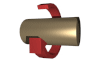NewY:=Pcos * Y - Psin * Z
NewZ:=Psin * Y + Pcos * Z

If you were looking down the +ve end of the X-axis, to rotate the point anti-clockwise (negative) around the axis we will use.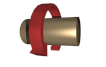NewY:=Ncos * Y - Nsin * Z
NewZ:=Nsin * Y + Pcos * Z

To rotate in the Y-axis the Y co-ordinate will not change so as like before the X and Z co-ordinate will change.
If you were looking down the +ve end of the Y-axis, to rotate the point anti-clockwise (positive) around the axis we will use.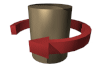NewX:=Pcos * X - Psin * Z
NewZ:=Psin * X + Pcos * Z

If you were looking down the +ve end of the Y-axis, to rotate the point clockwise (negative) around the axis we will use.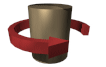NewX:=Ncos * X - Nsin * Z
NewZ:=Nsin * X + Pcos * Z

To rotate in the Z-axis the Z co-ordinate will not change so as like before the X and Y co-ordinate will change.
If you were looking down the +ve end of the Z-axis, to rotate the point anti-clockwise (positive) around the axis we will use.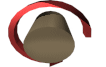NewY:=Pcos * Y - Psin * X
NewX:=Psin * Y + Pcos * X

If you were looking down the +ve end of the X-axis, to rotate the point anti-clockwise (negative) around the axis we will use.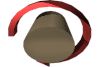NewY:=Ncos * Y - Nsin * X
NewX:=Nsin * Y + Pcos * X

Where the variable X, Y, and Z are the original X, Y, and Z values, and NewX, NewY, and NewZ are the new X, Y, and Z values for the point.

Now you have a point that can be moved in any axis, as many degrees as you want, CONGRATULATIONS, you are now programming in 3D. Not as hard as you originally thought it was going to be hey!!

Well to tell you the truth, you have not completely finished. If you where to do this now you will find that it is impossible to tell if the point was at the back of the screen or the front, and therefore you will not be able to tell which way the point is rotating, apart from the Z axis obviously.

Well to get around this all you need to do is use a bit of colour, like I did in my examples, GRID.PAS, F.PAS, and BOX.PAS. Just decrease the colour of the pixel, line, or point as the points Z co-ordinate gets further away from you, i.e. it becomes less, and increase its colour as it the Z value gets higher i.e. towards you.

Hint: You will notice how the 5CUBES demo makes the cubes look solid. Easy just don t display the lines of the box if there Z value is less than zero (cube faces are flat! ;-) ). There is a even better way. Below I have allowed you to download a program written in FPK-Pascal, one of the best if not the best Pascal 32bit compilers. Because of the old 64Kbyte limit is overcome in 32bit, the program below has been written to be able to load in 3D studio ASCII files, i.e. more complex objects than that of the previous examples. The code also incorporates write frame, solid and flat shading. Take a look once you have mastered the above algorithm.

I have given a compiled version in case you do not have FPK Pascal. Bare in mind that this compiled program will only work on an Intel Processor as it has been optimised to use this type of processor.

Well that's about it !!, I can still be contacted by e-mail if you are still having troubles, or if you think that the tutorial was not explanatory enough (I tried to make as explanatory as possible, I am sorry if you can not still understand what I was waffling on about !)

In the example I have given you at the top of this web page, I have incorporated Z perspective. This gives any object a kind of fish bowl look. Work out how it is done in the source, you will then have a better understanding!!

Enjoy,